# R S AGGARWAL AND V AGGARWAL Solutions for Class 9 Maths Chapter 19 - Probability

Page / Exercise

## Chapter 19 - Probability MCQ

Solution 1

Correct option: (d)

Total number of people = 645

Number of people having high school certificate = 516Solution 2

Correct option: (c)

Total number of students = 11 + 15 + 8 + 6 = 40

Number of students having blood group AB = 8Solution 3

Correct option: (d)

Total number of bulbs = 80

Number of bulbs having life of 1150 hours = 0

Required probability = 0

Solution 4

Correct option: (c)

Total number of children = 364

Number of children who like to eat potato chips = 91

Number of children who do not like to eat potato chips = 364 - 91 = 273Solution 5

Correct option: (b)

Total number of outcomes = 1000Solution 6

Correct option: (b)

Total number of bulbs = 80Solution 7

Correct option: (c)

Total number of students = 200

Number of students who does not like Sanskrit = 65Solution 8Solution 9Solution 10Solution 11Solution 12Solution 13Solution 14Solution 15Solution 16

Correct option: (c)

Total number of outcomes = 600## Chapter 19 - Probability Ex. 19

Solution 1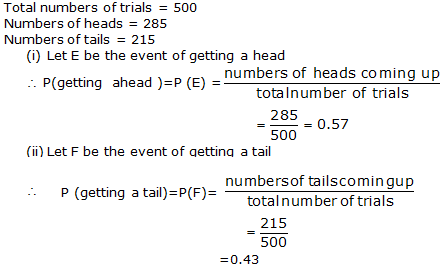Solution 2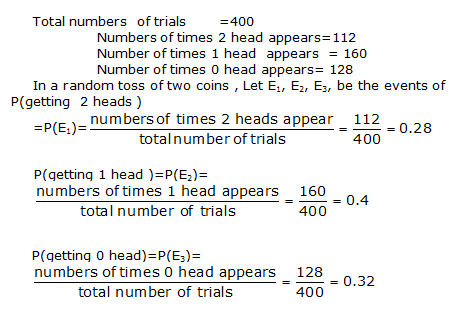Solution 3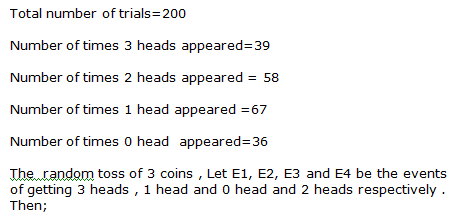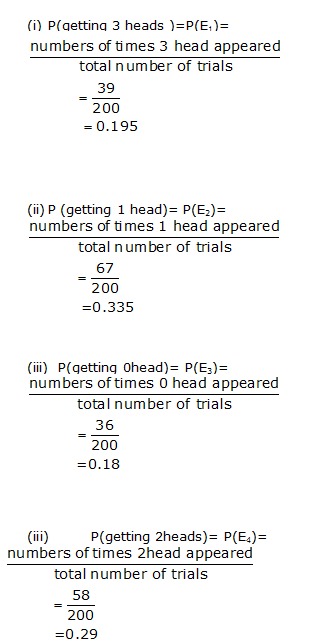Solution 4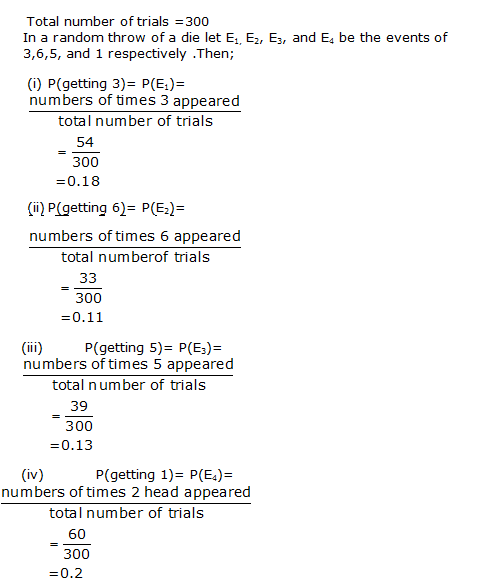Solution 5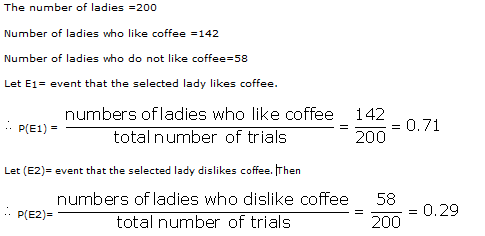Solution 6

Number of tests in which he gets more than60% marks =2

Total numbers of tests =6Required probabilitySolution 7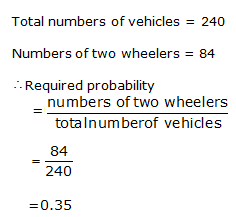Solution 8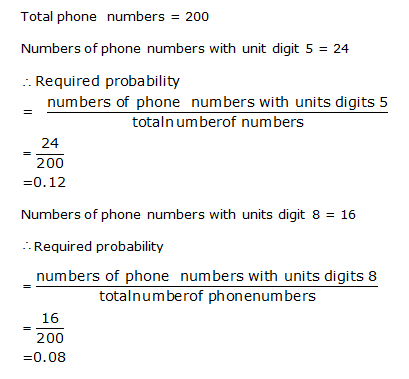Solution 9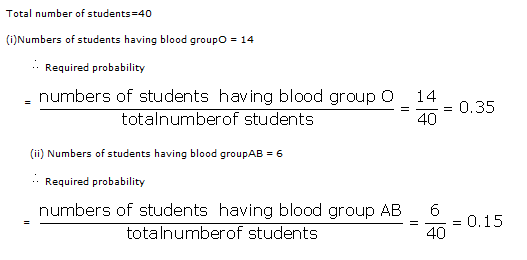Solution 10

Total number of salt packets = 12

Number of packets containing more than 2 kg of salt = 5

Therefore,

Probability that the chosen packet contains more than 2 kg of saltSolution 11

Total number of ball played = 30

Number of times boundary was hit = 6

Number of times boundary was not hit = 30 - 6 = 24

Therefore,

Probability that the batsman did not hit the boundarySolution 12Solution 13Solution 14Solution 15Solution 16Solution 17

Total number of electric bulbs = 800

Number of defective bulbs = 36

Number of non-defective bulbs = 800 - 36 = 764

Hence, probability that the bulb chosen is non-defectiveSolution 18

Fill in the blanks.

(i) Probability of an impossible event = 0

(ii) Probability of a sure event = 1

(iii) Let E be an event. Then, P(not E) = 1 - P(E)

(iv) P(E) + P(not E) = 1

(v) 0 ≤ P(E) ≤ 1

### STUDY RESOURCES

REGISTERED OFFICE : First Floor, Empire Complex, 414 Senapati Bapat Marg, Lower Parel, Mumbai - 400013, Maharashtra India.